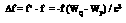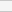GNSS Geodesy Innovation Interviews UAV/UAS Positioning Surveying Applications Perspective Time Navigation Mapping Policy Land Administration News Archives LBS GIS SDI Imaging E-ZineGeodesy

# Measuring geopotential difference between two points

Dec 2005 | Comments Off on Measuring geopotential difference between two points

###### By receiving the light signals emitted by a light source simultaneously at two points P and Q located on the Earth’s surface, it is possible to directly measure the geopotential difference between P and Q, based on the gravity frequency shift equation

Conventionally, the potential difference between two points P and Q located on the Earth’s surface are determined by gravimetry and levelling (Heiskanen and Moritz 1967), the drawback of which is that it is almost impossible to connect these two points in the case that they are located on two continents, because it is well known that the potential surface of the mean sea level (MSL) is not an equipotential surface. In another aspect, if given the gravity data on the Earth’s surface, one might determine the potential difference between two points by using the Stokes method or Molodensky method (ibid). In this case the potential field is determined and consequently the potential difference between two arbitrary points could be determined. However, Stokes method is approximate due to the requirement that the measured gravity data should be deduced on the geoid, which gives rise to obvious errors; Molodensky method is complicated, and the determined is the quasi-geoid. The transformation from quasi-geoid to geoid needs again levelling, which encounter difficulties in connecting two points located on different continents. Hence, applying the conventional approach, it is very dif?cult to establish a unified global datum system (with adequate precision). To avoid this difficulty, Bjerhammar (1985) suggested that the geopotential difference between two arbitrary points P and Q might be determined by using two precise clocks put at P and Q respectively, based on the theory of general relativity (e.g. Weinberg 1972). The basic principle is stated as follows. According to Einstein’s general relativity theory, the running rate of a clock is closely related with the geopotential at the point where the clock is located: the clock located at the position with higher geopotential runs faster than the clock located at the position with lower geopotential. Based on the above considerations, Bjerhammar defined a relativistic geoid as follows: the relativistic geoid is the equipotential surface nearest to MSL on which a precise clock runs with the same rate. This is referred to as the equi-time-rate geoid (Shen 1998).

Different from Bjerhammar, Shen et al(1993) argued that it is better to determine the geopotential difference by receiving the light signals emitted by an emitter (which could be located on the Earth’s surface or in space), based on the gravity frequency shift equation, which is not only the result derived from the general relativity but also the quantum mechanics and energy conservation law (Shen 1998). Further, it was shortly proposed that (Shen et al 1993; Shen 1998), it might be possible to directly determine the potential difference between two points P and Q on the surface of the Earth (even these two points are located on different continents) by receiving the light signals emitted by a satellite or a distant star.

Suppose an emitter is equipped with a fiying satellite, which can emit light signals with regular intervals. Then, by receiving the light signals emitted by the emitter simultaneously at two points P and Q, one could determine the geopotential difference between P and Q, based on the gravity frequency shift principle. Then, one can determine the potential field based on the truncated spherical harmonic expansion combining with the least squares adjustment (e.g., Rummel et al 1993) or the fictitious compress recovery approach (Shen 2004), the main idea of the latter is stated as follows. Compress the boundary value, given on the Earth’s surface, on the surface of an inner sphere located inside the Earth, and using Poisson integral one gets a harmonic field in the domain outside the inner sphere; compress again the residuals between the initial boundary value and the calculated one, provided on the Earth’s surface, on the surface of the inner sphere, and using Poisson integral one gets again a harmonic field in the domain outside the inner sphere; this procedure is repeated and a series result is obtained, which coincides with the real field in the domain outside the Earth.

The gravity frequency shift principle is stated as follows (Shen et al. 1993; Shen 1998).

Suppose a light signal with frequency f is emitted from point P by an emitter that is not necessarily located on a satellite, and the signal is received at point Q by a receiver (Cf. Fig.1). Because of the geopotential difference between these two points, the frequency of the received light signal is not f but f’. Using fP and fQ to denote f and f’ respectively, the following equation holds (Pound and Snider 1965; Shen et al. 1993):where c is the velocity of light in vacuum, WP and WQ are the geopotentials at points P and Q respectively. Expression (1) is referred to as the gravity frequency shift equation (Pound and Snider 1965), or properly called the geopotential frequency shift equation due to the fact that the frequency shift is caused by the geopotential difference. Katila and Riski (1981) con?rmed Eq. (1) with accuracy 10-2. Vessot et al.

1) with accuracy 10-2. Vessot et al. (1980) proved that Eq. (1) is correct to the accuracy of 10-4. Scientists believe that Eq. (1) is correct, because it is a result derived from the theory of general relativity. As mentioned previously, Eq. (1) can be also derived out based on quantum theory and energy conservation law (Shen, 1998). Hence, suppose the geopotential at point P is given, then, from Eq. (1) the geopotential at an arbitrary point Q can be determined by measuring the geopotential frequency shift between P and Q.

Set at point P an emitter which emits a light signal with frequency f and a receiver at point Q receives the light signal emitted by the emitter at point P. Suppose the received signal’s frequency is f’. Then, it could be compared the frequency f’ of the received light signal with it self’s standard frequency f (this is not only the emitting frequency at point P but also the standard innate frequency of the receiver at point Q), and the frequency shift fif = f’ – f can be determined. Consequently, according to Eq. (1) the geopotential difference fiWPQ between P and Q
can be determined. Applying the same principle it will be found the geopotential difference fiWOP = WP – WO between the point O on the geoid and an arbitrarily point P, where WO = C0 is the geoid geopotential constant. If C0 is a known constant, WP as well as WQ can be determined. Hence, based on the geopotential frequency shift equation (1), the relativistic geoid could be defined as follows (Shen et al 1993): the relativistic geoid is the equi-potential surface nearest to MSL on which there does not exist light signal’s

«Previous 1 2View All| Next»

Pages: 1 2(No Ratings Yet)Loading...